# Научный форум dxdy

Математика, Физика, Computer Science, Machine Learning, LaTeX, Механика и Техника, Химия,
Биология и Медицина, Экономика и Финансовая Математика, Гуманитарные науки

 Печатать страницу | Печатать всю тему Пред. тема | След. тема

 Re: Al Zimmermann: Primorial Soup04/10/17

153
 Последний раз редактировалось as73251 26.12.2017, 16:42, всего редактировалось 1 раз. 12d3Спасибо. А какие Et и Best Raw Score Вы использовали при генерации набора для n=13? Ведь приведенное решение для n=13 почти наверняка неоптимальное.Re: Al Zimmermann: Primorial Soup18/11/10
75
 Последний раз редактировалось Jarek 26.12.2017, 16:46, всего редактировалось 1 раз. as73251 в сообщении #1278922 писал(а):Это я понимаю: я имел в виду, будете ли Вы менять свой подход - ведь Al ждет прежде всего оптимальных или близких к оптимальным решения. А жаль: Ваш талант позволил бы Вам продвинуться и по другим направлениям.I am following discussion on this forum and of course I can contribute ideas if I see I have anything useful to say. However, I believe that as far as exhaustive search methods are considered, other people can do better than I. So I do not expect to be of much help here.My approach protected me from finding an optimal solution. Here is why. Statistically, one should expect that in optimal solution small primes should be distributed more or less randomly, i.e. they shouldn't meet frequently at the same vertex. My approach assumed the contrary: I placed a few small primes at the same vertex to move them out of my way before I reach last vertices.Re: Al Zimmermann: Primorial Soup
 Заслуженный участник04/03/09
897
 as73251 в сообщении #1278930 писал(а):А какие Et и Best Raw Score Вы использовали при генерации набора для n=13?Я пробовал и то, что было в табличке на тот момент, и раза в два-три-четыре меньше (потому что по моим оценкам, оптимальное решение должно быть меньше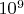$10^9$. Все равно мой алгоритм не позволил найти решение для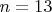$n=13$.Re: Al Zimmermann: Primorial Soup04/10/17

153
 Последний раз редактировалось as73251 26.12.2017, 16:57, всего редактировалось 1 раз. 12d3 в сообщении #1278936 писал(а):as73251 в сообщении #1278930 писал(а):А какие Et и Best Raw Score Вы использовали при генерации набора для n=13?Я пробовал и то, что было в табличке на тот момент, и раза в два-три-четыре меньше (потому что по моим оценкам, оптимальное решение должно быть меньше$10^9$. Все равно мой алгоритм не позволил найти решение для$n=13$.А жаль: ваш вклад в исследование данной задачи достоин большего, чем вы достигли в конкурсе.-- 26.12.2017, 16:57 --JarekВы явно скромничаете. Что касается Вашего подхода, то я внимательно ознакомился с ним в Discussion Group.Re: Al Zimmermann: Primorial Soup10/05/09
66
Москва
 Последний раз редактировалось Skrejet 27.12.2017, 06:51, всего редактировалось 1 раз. Оформил генетический алгоритм для n-хромосомных генотипов, с весами соединений вершин, соответствующих каждой из хромосом. При некоторых корректировках параметров запуска алгоритм достаточно быстро сходится в область решений с малым относительным отклонением энергий от оптимальной оценки при любых n (время сходимости в среднем растет от n неочень круто), но решения получаются довольно грубыми, после чего эволюция резко замедляется, так что сразу непонятно насколько сошелся алгоритм. Кроме того промежуточные решения на этапе сходимости не улучшаются элементарными вариацями, причем при больших n они оказываюся довольно далеки от оптимальных, а чтобы можно было зацепиться за очки нужны были более-менее точные приближения. Алгоритм работает без начальных приближений, но для эффективности поиска после поколений первичной сходимости скорее всего нужны другие модификации опереторов - не хватает комбинированных мутаций и мутационных стратегий для их управления.Re: Al Zimmermann: Primorial Soup01/11/17
40
 Skrejet в сообщении #1279096 писал(а):Оформил генетический алгоритм ...Hi Skrejet,I did similar attempts.Actually this way I composed my lists of best nodes. Starting from sufficiently unbiased seed, remove n primes (in all possible ways), than add n primes (in all possible ways) and store if the product of primes is sufficiently low to fit in a list of predefined number of best nodes. For the graph of size 12, n=3 resulted in enclosed list which n=4 for hours didn't improve even with a single node. For graph of size 13 mutation at depth 4 helped up to some degree, but I didn't finished it completely.My internal representation of the graph is an ordered set of prime indexes. I did it in this way intentionally to be able to swap safely indexes being sure that always a valid graph is composed. (In addition I took some care to morph the reproduced graph to its canonical form). Again, I blindly did taking all possible combinations of n primes and permuting them in all possible ways (early stopping when obviously some product/node increases too much). This worked with very limited success, as you said, resulting in some "good" graphs which later turned out to be not so good. For the large graphs I hoped that the space becomes more smooth and this could work. Nope. Barriers between the local minima are still too high.However the exchange of the edges between small subset of nodes worked. Surprisingly, as other mentioned, none of the best graphs was discovered in this way.To clarify what I meant by "exchange of edges between nodes". A graph is easy to decompose to 2 pieces by dividing the nodes into 2 groups and cutting the edges between one group and another.For simplicity let one group consists from nodes (A,B) and the other from the rest nodes (C,D,E,F). We are keeping the edge between A and B as well as all edges between the nodes in the second group. We are cutting 2 edges connecting (A-C) and (B-C) and re-connecting them by exchanging A and B so that the first edge now connects (B-C) and the second (A-C).The above mutation left nodes (C,D,E,F) unchanged (i.e. the product of the primes connected to them) and changes only nodes A and B. For all the possible such mutations (with also edges to D,E,F involved), one gives minimality for sum(weight(A), weight(B)) and leaves the rest nodes unchanged.The same is applicable for partitioning the same graph to (A,B,C) union (D,E,F), etc.I ran code for exhaustive rebuilding of subgraphs of size 3 for thousands of graphs, and up to 4 for the "top" graphs in my cache.On the other end, I ran code that takes all possible node triplets from a given good graph, and rebuilds graphs (of size 13 to 15) by appending nodes from a list of known good nodes. Again, this resulted in generation of significant amount of good graphs which "surprisingly" were not better than the seed used.Since these mutations are symmetrical, my explanation is the bias of the seed originating from the previously applied transformations and filtering.Re: Al Zimmermann: Primorial Soup25/08/12
167
Germany
 Последний раз редактировалось Herbert Kociemba 27.12.2017, 23:09, всего редактировалось 2 раз(а). I still are interested in the moment more in the task to estimate the best possible rawscores and not to find solutions. I am quite satisfied that Jarek also uses the "logarithmic view" of the problem which has some advantages in my opinion.Here are some formulas which are worth to be mentioned, some of them I have posted already before. Let n be the problem size.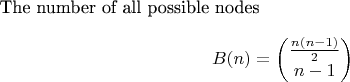$The number of all possible nodesB(n)=\begin{pmatrix}\frac {n(n-1)} 2\\n-1\end{pmatrix}$$The probability that adding a random node from the set of all possible nodes to a list of k compatible nodes gives a list of k+1 compatible nodes p(n,k)=\frac {(n-k)^kB(n-k)}{B(n)}, \text{ } k = 0...n-1$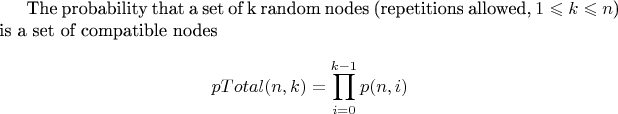$The probability that a set of k random nodes (repetitions allowed, 1\leqslant k \leqslant n) is a set of compatible nodes pTotal(n,k)=\prod_{i=0}^{k-1}p(n,i)$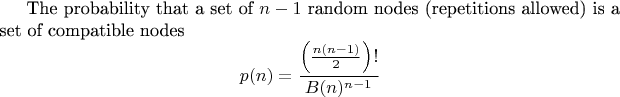$The probability that a set of n-1 random nodes (repetitions allowed) is a set of compatible nodes p(n)= \frac{\left(\frac{n(n-1)}{2}\right)!}{B(n)^{n-1}}$$p(n)$ should be - and is -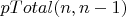$pTotal(n,n-1)$ but the identity is not obvious at all.$The energy of a node (logarithmic)E=\sum_{prime \in node} \ln(prime)$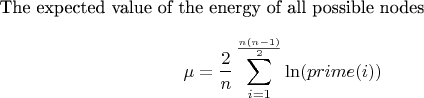$The expected value of the energy of all possible nodes\mu = \frac{2}{n}\sum_{i=1}^{\frac{n(n-1)} 2} \ln(prime(i))$This is also the target energy for a node.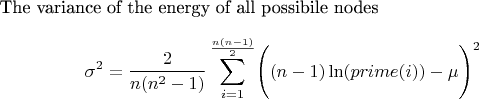$The variance of the energy of all possibile nodes \sigma^2 = \frac{2}{n(n^2-1)} \sum\limits_{i=1}^{\frac{n(n-1)} 2}\Biggl((n-1)\ln(prime(i))-\mu\Biggr)^2$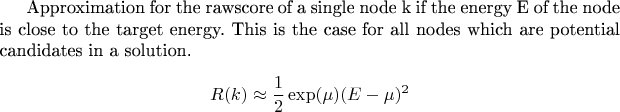$Approximation for the rawscore of a single node k if the energy E of the node is close to the target energy. This is the case for all nodes which are potential candidates in a solution. R(k)\approx \frac 1 2 \exp(\mu)(E-\mu)^2$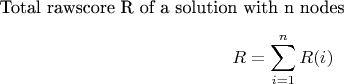$Total rawscore R of a solution with n nodes R= \sum_{i=1}^n R(i)$$Number of nodes with energies in the interval [\mu-\varepsilon,\mu+\varepsilon], \varepsilon\ll1 N(n,\varepsilon)\approx \frac{2 \varepsilon B(n)} {\sqrt{2\pi\sigma^2}}$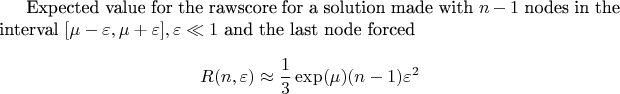$Expected value for the rawscore for a solution made with n-1 nodes in the interval [\mu-\varepsilon,\mu+\varepsilon], \varepsilon\ll1 and the last node forced R(n,\varepsilon)\approx \frac 1 3 \exp(\mu)(n-1)\varepsilon^2$With this framework of formulas it is possible to estimate the best possible rawscores. If anybody should be interested in the derivation of a formula I can provide it.Re: Al Zimmermann: Primorial Soup01/06/12
1006
 Happy New Year everyone! I wish that 2018 brings you much success and many new discoveries!Re: Al Zimmermann: Primorial Soup09/03/16
34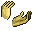dimkadimon в сообщении #1280348 писал(а):Happy New Year everyone! I wish that 2018 brings you much success and many new discoveries!Re: Al Zimmermann: Primorial Soup25/08/12
167
Germany
 Since I looked at the problem in an additive/logarithmic way it took only small modifications of my program to handle the following problem. Given a complete graph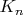$K_n$, number the edges in such a way with the numbers 1...n(n-1)/2 that the sum of the edge weights is the same for each vertex. It is well known that except for the trivial case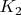$K_2$ this is possible exactly for all n>=6 with n<>0 mod 4. According to https://en.wikipedia.org/wiki/Magic_graph we can call this a supermagic (complete) graph.I wanted to know for which n there exist bi-supermagic complete graphs. This means that not only the sum of the edge weights for each vertex is constant but also the sum of the squared edge weights. I found that this is not possible until n>9. For n=10 there are already lots of them and they are quite easy to find. The picture shows an example. The magic constant is 207 for the sum of the edge weights and 6279 for the sum of the squared edge weights. The color of the edges corresponds to the weights - if the weights are close together so are the corresponding colors.Re: Al Zimmermann: Primorial Soup01/06/12
1006
 Herbert Kociemba, great work! Very pretty picture too. I wonder if the same is possible when the edge weights are primes?Re: Al Zimmermann: Primorial Soup04/10/17

153
 Последний раз редактировалось as73251 30.04.2018, 14:39, всего редактировалось 1 раз. Модифицировал свой алгоритм с целью нахождения только оптимальных решений: для n=12 время счета менее трех минут на одном ядре.Показать сообщения за: Все сообщения1 день7 дней2 недели1 месяц3 месяца6 месяцев1 год Поле сортировки АвторВремя размещенияЗаголовок по возрастаниюпо убыванию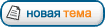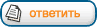Страница 12 из 12 [ Сообщений: 177 ] На страницу Пред.  1 ... 8, 9, 10, 11, 12

Модераторы: maxal, Toucan, PAV, Karan, Супермодераторы

#### Кто сейчас на конференции

Сейчас этот форум просматривают: нет зарегистрированных пользователей

 Вы не можете начинать темыВы не можете отвечать на сообщенияВы не можете редактировать свои сообщенияВы не можете удалять свои сообщенияВы не можете добавлять вложения

 Найти: